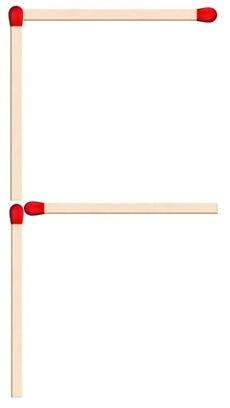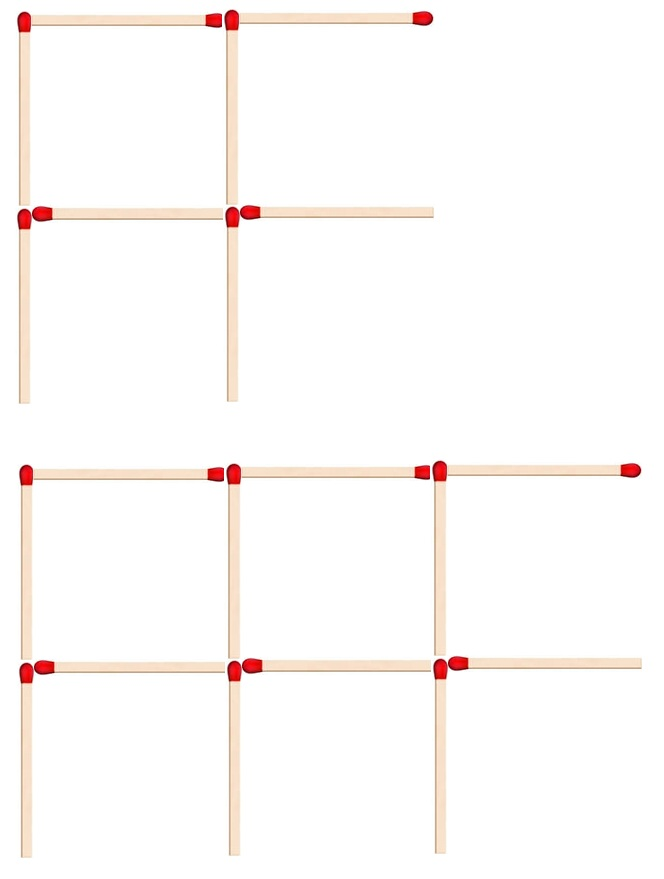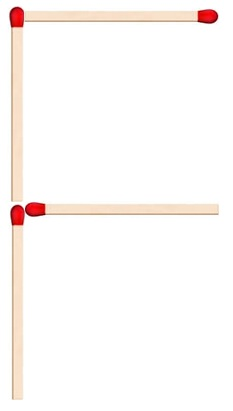Matchstick pattern

Chapter 11 Class 6 Algebra
Concept wise

Suppose we use matchsticks to make letter FHere, we used 4 matchsticks

Suppose, we want to Make more than one Fs.So,

2 Fs require 8 matchsticks

3 Fs require 12 matchsticks

4 Fs require 16 matchsticks

and so on……So,

we can say that

Number of matchsticks = 4 × Number of Fs

Writing n = number of Fs

Number of matchsticks = 4n

Let’s check

For n = 1, Number of matchsticks = 4 × 1 = 4

For n = 2, Number of matchsticks = 4 × 2 = 8

For n = 3, Number of matchsticks = 4 × 3 = 12

So, writing

Number of matchsticks = 4 n

makes our job easier

Suppose we have to make 100 Fs

Number of matchsticks = 4 × 100

= 400

Learn in your speed, with individual attention - Teachoo Maths 1-on-1 Class